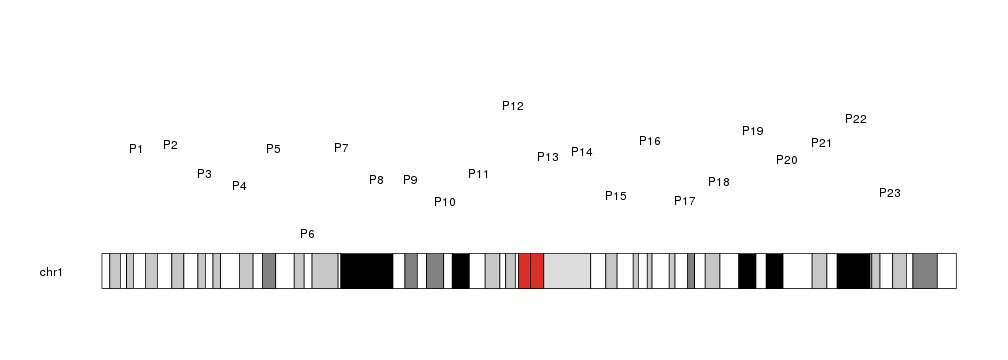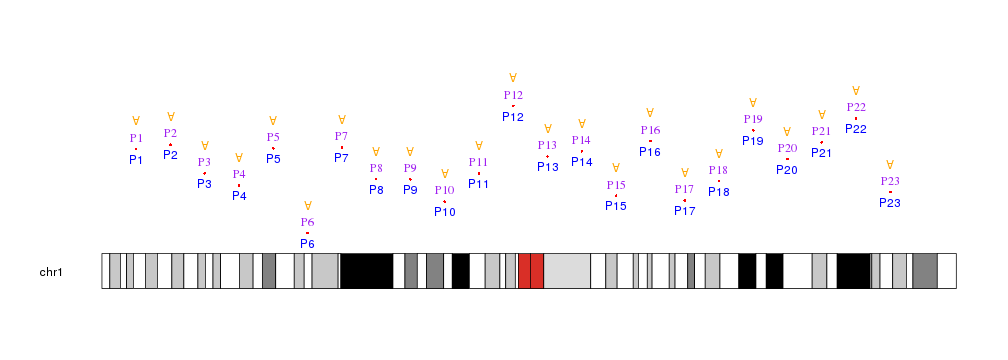Plotting Text

To plot Text in a karyoplot we need to use the kpText function. Given a character vector chr, two integer vectors x and y and a character vector labels, it will plot the text in labels in the positions defined by chr, x and y.

library(karyoploteR)

x <- 1:23*10e6
y <- rnorm(23, mean=0.5, sd=0.25)
labels <- paste0("P", c(1:23))

kp <- plotKaryotype(chromosomes="chr1")
kpText(kp, chr="chr1", x=x, y=y, labels=labels)Text are cutomizable with the same graphical parameters as in the R base graphics text function: cex, pos, srt, col

kp <- plotKaryotype(chromosomes="chr1")
kpPoints(kp, chr="chr1", x=x, y=y, col="red")
kpText(kp, chr="chr1", x=x, y=y, labels=labels, col="blue", pos=1)
kpText(kp, chr="chr1", x=x, y=y, labels=labels, col="purple", family="serif", pos=3)
kpText(kp, chr="chr1", x=x, y=y+0.2, labels="A", col="orange", srt=180)